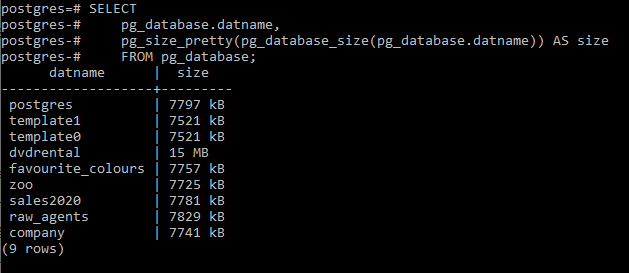Open In App

# PostgreSQL – Size of a Database

In this article, we will look into the function that helps us to evaluate the size of a given database. The` pg_database_size()` function is used to get the size of a database.

`Syntax: select pg_database_size('database_name');`

Now let’s list all the available database available on our server and find their sizes in our example using the below command:

`\l`

This gives us the following result: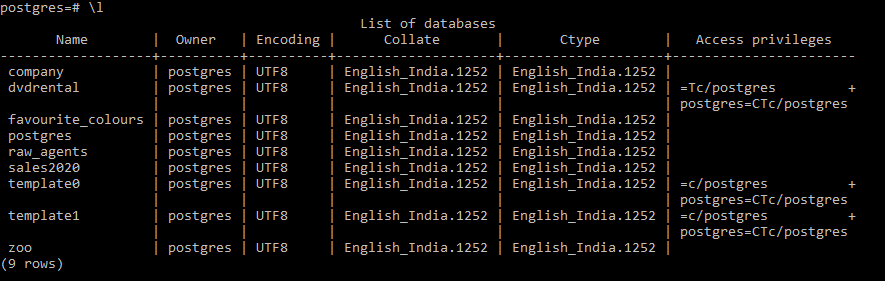Example 1:
Here we will query for the size of the dvdrental database in our server using the below command:

`select pg_database_size('dvdrental');`

Output: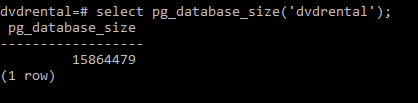To make the result readable, one can use the `pg_size_pretty()` function. The pg_size_pretty() function takes the result of another function and format it using bytes, kB, MB, GB or TB as required. So the above output can be modified as below:

```SELECT
pg_size_pretty (
pg_database_size ('dvdrental')
);```

Output: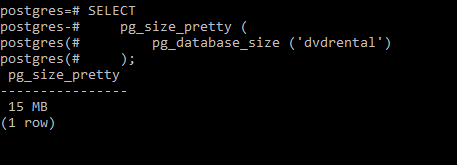Example 2:
Here we will query for the size of the zoo database in our server using the below command:

```SELECT
pg_size_pretty (
pg_database_size ('zoo')
);```

Output: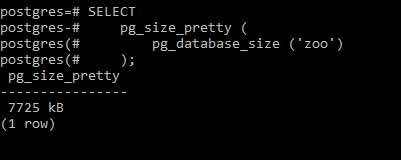Example 3:
Here we will query for the size of the sales2020 database in our server using the below command:

```SELECT
pg_size_pretty (
pg_database_size ('sales2020')
);```

Output: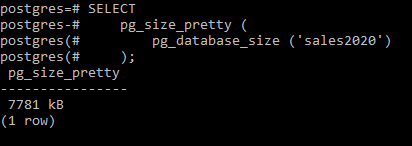Example 4:
Here we will query the size of every database in our current server using the below command:

```SELECT
pg_database.datname,
pg_size_pretty(pg_database_size(pg_database.datname)) AS size
FROM pg_database;```

Output: